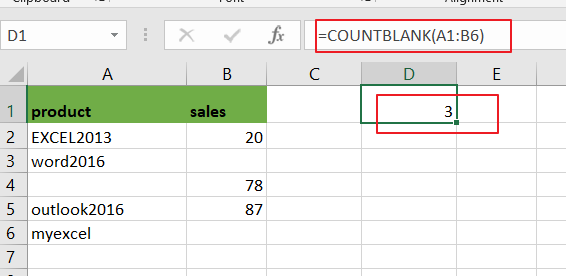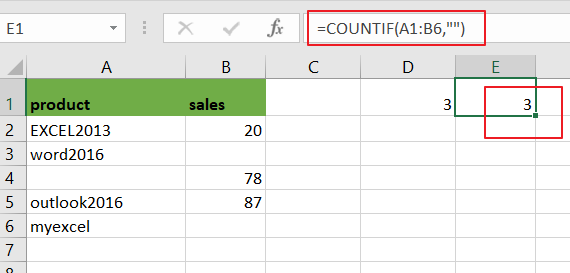# How to Count Blank or Empty Cells in Excel

This post will guide you how to count blank or empty cells in a given range cells using a formula in Excel 2013/2016.How do I count the number of cells that are blank in Excel. You should know that excel offers several count functions to count the number of cells in a selected range of cells that match a criteria, such as: containing a specific text string or letter.

If you want to count the number of cells in a selected range that do not contain any data values, you can use the COUNTBLANK function.

## Count Blank or Empty Cells

Assuming that you want to do a quick count of empty cells in a selected range(A1:A6).In this case, you can use a formula based on the COUNTBLANK function to obtain the empty cells.

=COUNTBLANK(A1:B6)To use this above formula, you just need to type it on the formula space. And you also need to change the cells you need to obtain a count on in the above formula.

As you can see in the above screenshot, the number of cells that are blank from range A1:B6 is three in that case.

Now Let’s see how this formula works:

The COUNTIFBLANK function can be used to count the number of cells in a selected range that don’t contain any value. It means that cells that contain text, numbers, errors, etc. are not counted.

You can also use another function called COUNTIF function to achieve the same result of counting the number of cells that are blank in Excel. For example, using the following formula:

=COUNTIF(A1:B6,””)From the above image, you would see that using the COUNTIF function to count the number of blank or empty cells in the range A1:B6 and return the same results as COUNTBLANK function.

### Related Functions

• Excel COUNTIF function
The Excel COUNTIF function will count the number of cells in a range that meet a given criteria. This function can be used to count the different kinds of cells with number, date, text values, blank, non-blanks, or containing specific characters.etc.= COUNTIF (range, criteria)…
• Excel COUNTBLANK function
The Excel COUNTBLANK function use to count the number of empty cells in a range of cells. So you can use the COUNTBLANK function to get the number of blank cells in a given range in Excel.The syntax of the COUNTBLANK function is as below:= COUNTBLANK (range)…